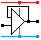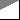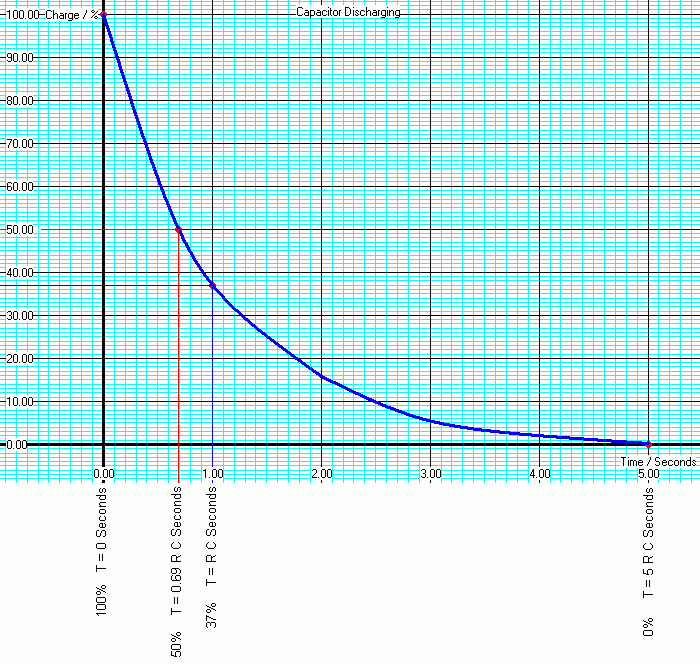Home

rOm
Quest
Glossary

Random
Page
Search
Site
Lush
Sim
Class
Subject
Images

Help
FAQ
Sign
Up
Log
In
 AS Level     >Timing<     RC Timing     Schmitt NOT Astable

# Timing

Site for Eduqas/WJEC - Go to the AQA site.

AS Level    RC TimingQuestions 0 to 3   -->  View All

Use a resistor and a capacitor to make a timing circuit.
Time Constant:   T   =   R C   =   The time for the capacitor to charge or discharge by 63%.

• Capacitors can be used, with a resistor, for timing.
• The capacitor acts a bit like a bucket of water.
• The resistor controls how fast the capacitor (bucket) fills or empties.
• The 555 timer uses RC combinations for timing.
• In this simulation, click the switch to the charging or discharging position.

View the RC timer in the Lushprojects Simulator

The time constant calculations below are needed for designing timing circuits.

• T is the time in seconds.
• R is the resistor value in Ohms.
• C is the capacitor value in Farads.

In this circuit, when the switch is flipped, the voltage across the capacitor changes quite fast and the current flowing is larger. As the capacitor approaches full charge (or discharge) the voltage changes more slowly and the current is smaller. The current is proportional to the rate of change of voltage.

Here is a Flash animation of a water analogy. The container represents the capacitor. It takes time to fill or empty. When the tank is nearly full or empty, the level changes much more slowly. Capacitors behave the same way.

## RC Timing Calculations

### ChargingR = 1000000 Ohms. C = 1 Microfarad. R C = 1 Second. 0% T = 0 Seconds 50% T = 0.69 R C Seconds 63% T = R C Seconds 66.6% T = 1.1 R C Seconds (555 Monostable) 100% T = 5 R C Seconds

### DischargingR = 1000000 Ohms. C = 1 Microfarad. R C = 1 Second. 100% T = 0 Seconds 50% T = 0.69 R C Seconds 37% T = R C Seconds 0% T = 5 R C Seconds

## Experiment

The aim is to measure the voltage across a charging capacitor at regular time intervals. Once the capacitor is fully charged, repeat the measurements while it is discharging. These results should be plotted on a graph. The graph should then be marked up with the key positions listed above.

• R = 47kΩ
• Charge for 10 minutes. Connect the resistor to the 5 Volt supply.
• Discharge for 10 minutes. Connect the resistor to the 0 Volt supply.
• Don't turn off the stopwatch at half time!• Calculate the time constant T = RC
• Note that capacitors are not usually manufactured with great accuracy and DC leakage may be noticeable when you take your measurements.

## Plot and Label the Graph

Charging ...

• Mark the graph at the 50% mark after 0.69RC seconds
• Mark the graph at the 63% mark after RC seconds
• Mark the graph at the 66.6% mark after 1.1RC seconds
• Mark the graph at the 100% mark after 5RC seconds

Discharging

• Mark the graph at the 50% mark after 0.69RC seconds
• Mark the graph at the 37% mark after RC seconds
• Mark the graph at the 0% mark after 5RC seconds

### Leakage

• Estimate the leakage resistance of the capacitor. (This may well not be constant.)

## Picoscope Traces for a Faster Timing Circuit

• R = 1 kΩ
• C = 0.47 µFSubject Name     Level     Topic Name     Question Heading     First Name Last Name Class ID     User ID

 CloseEscapeX Q: qNum of last_q     Q ID: Question ID         Score: num correct/num attempts         Date Done

Question Text

image url

Help Text
Debug

• You can attempt a question as many times as you like.
• If you are logged in, your first attempt, each day, is logged.
• To improve your scores, come back on future days, log in and re-do the questions that caused you problems.
• In the grade book, you can delete your answers for a topic before re-doing the questions. Avoid deleting unless you intend re-doing the questions very soon.